# RD Sharma Solutions for Class 11 Chapter 3 - Functions Exercise 3.3

In Exercise 3.3 of Chapter 3, we study the domain of real functions and range of real functions. Students who wish to learn correct methods of solving problems can have a quick reference to RD Sharma Class 11 Solutions. Solutions are developed by our experts to help students to follow the steps in correct methodology to easily crack the problems and score well in their board exams. The solutions to this exercise are provided in the easily available pdf format, which can be downloaded from the links provided below.

## Download the Pdf of RD Sharma Solutions for Class 11 Maths Exercise 3.3 Chapter 3 – Functions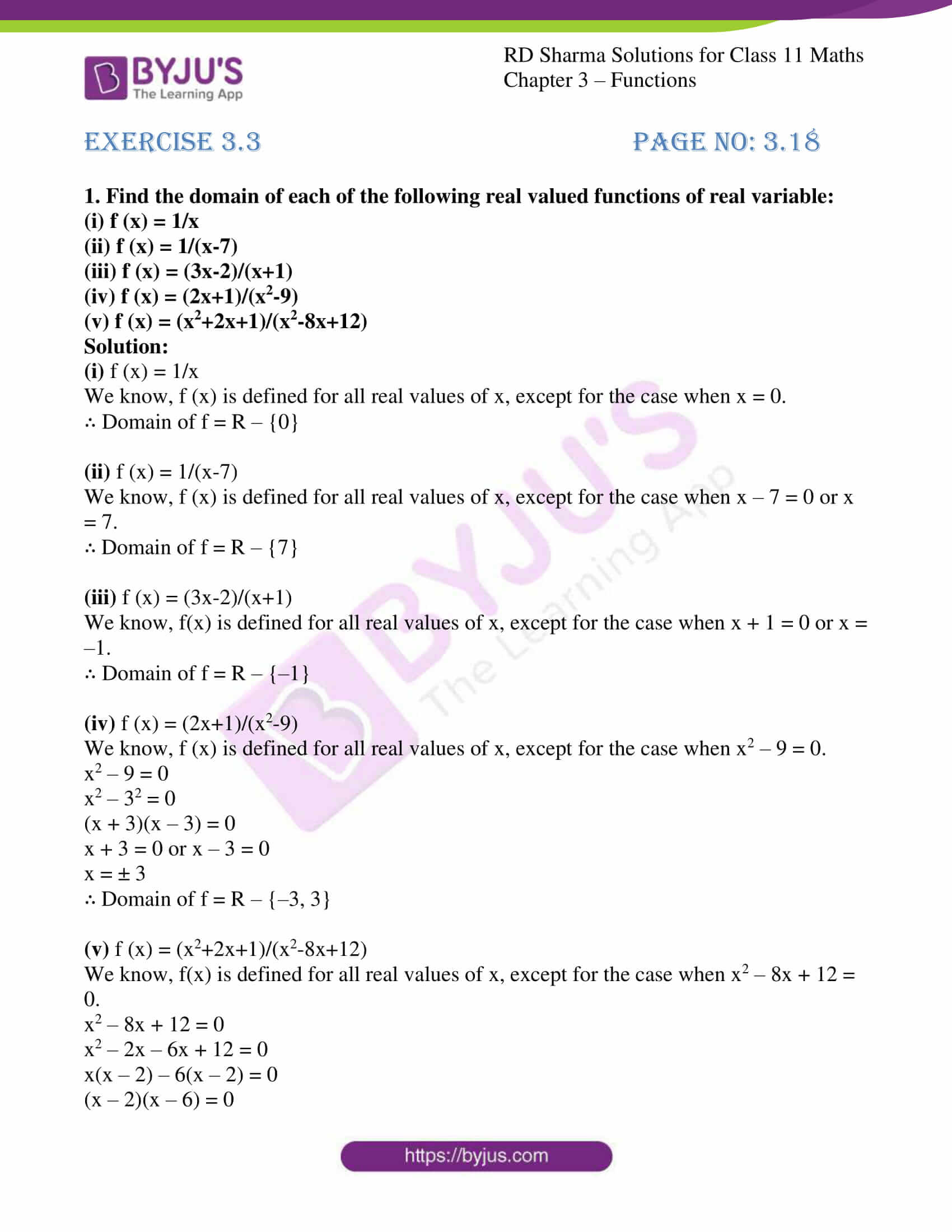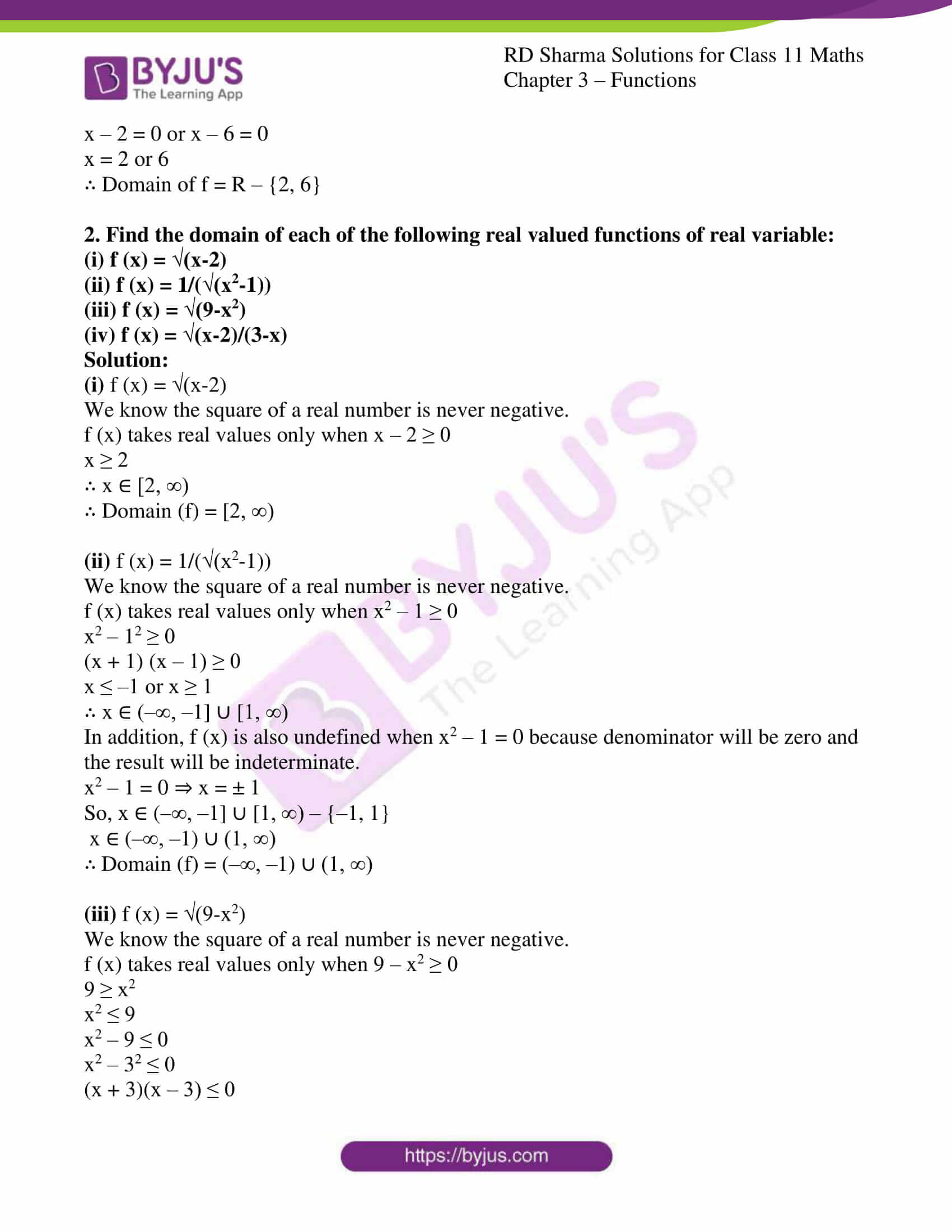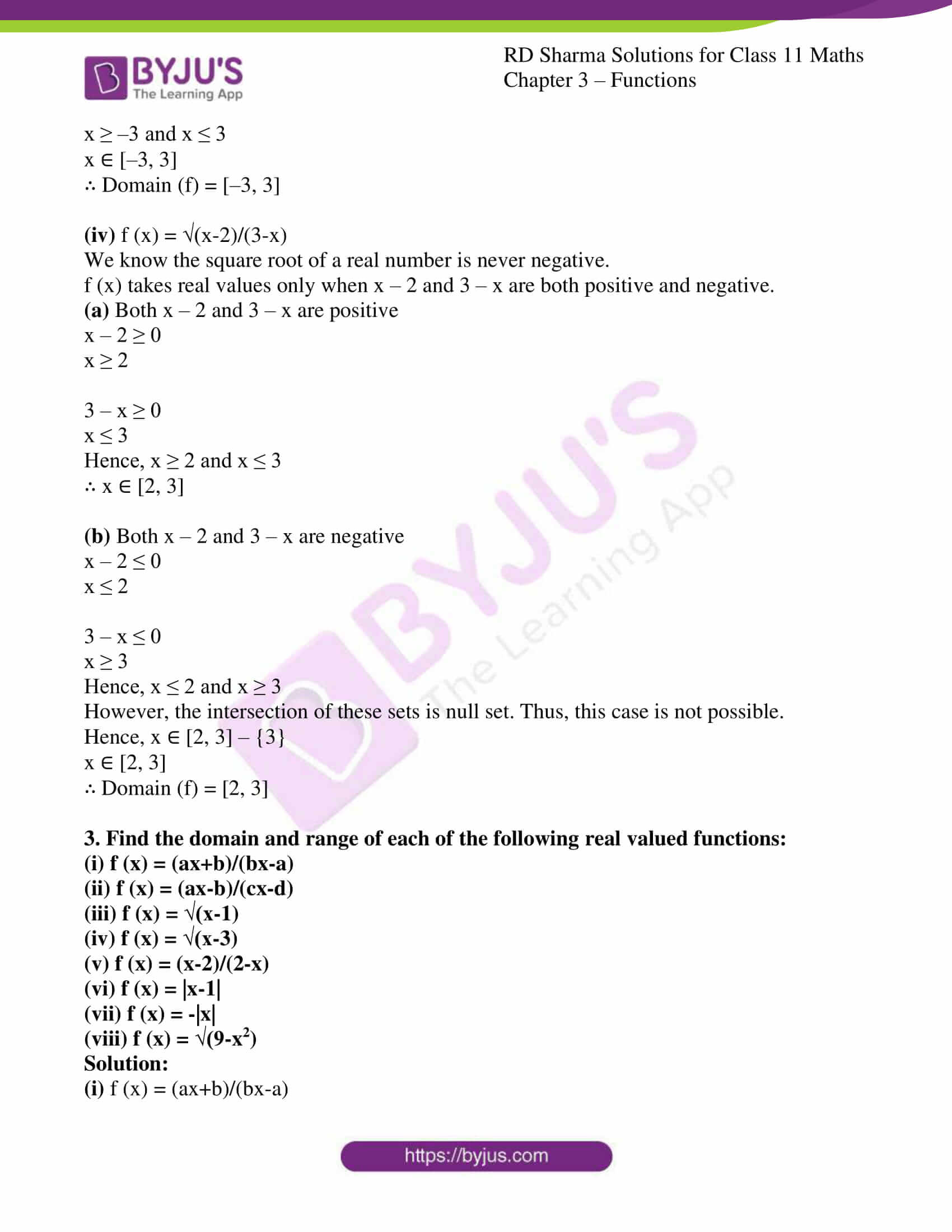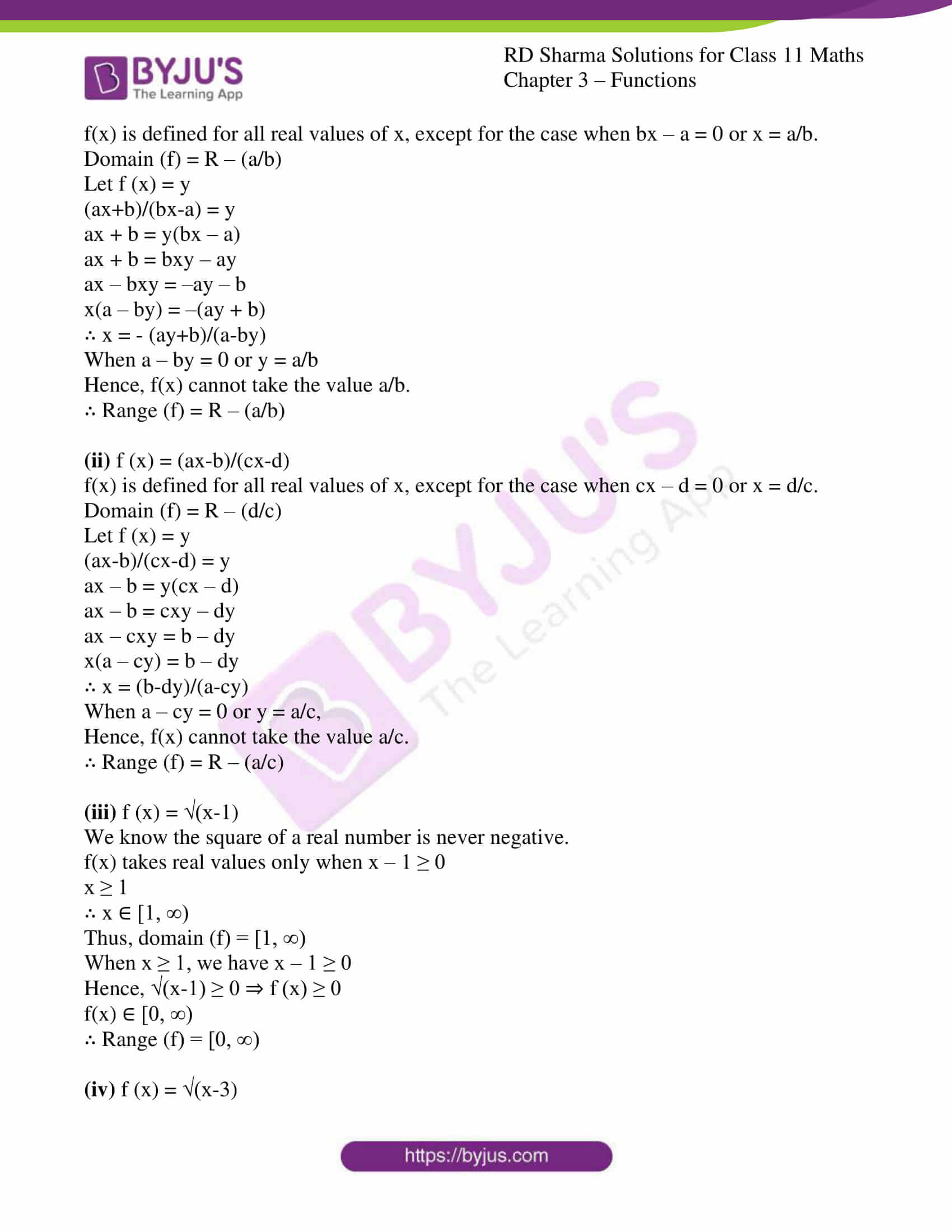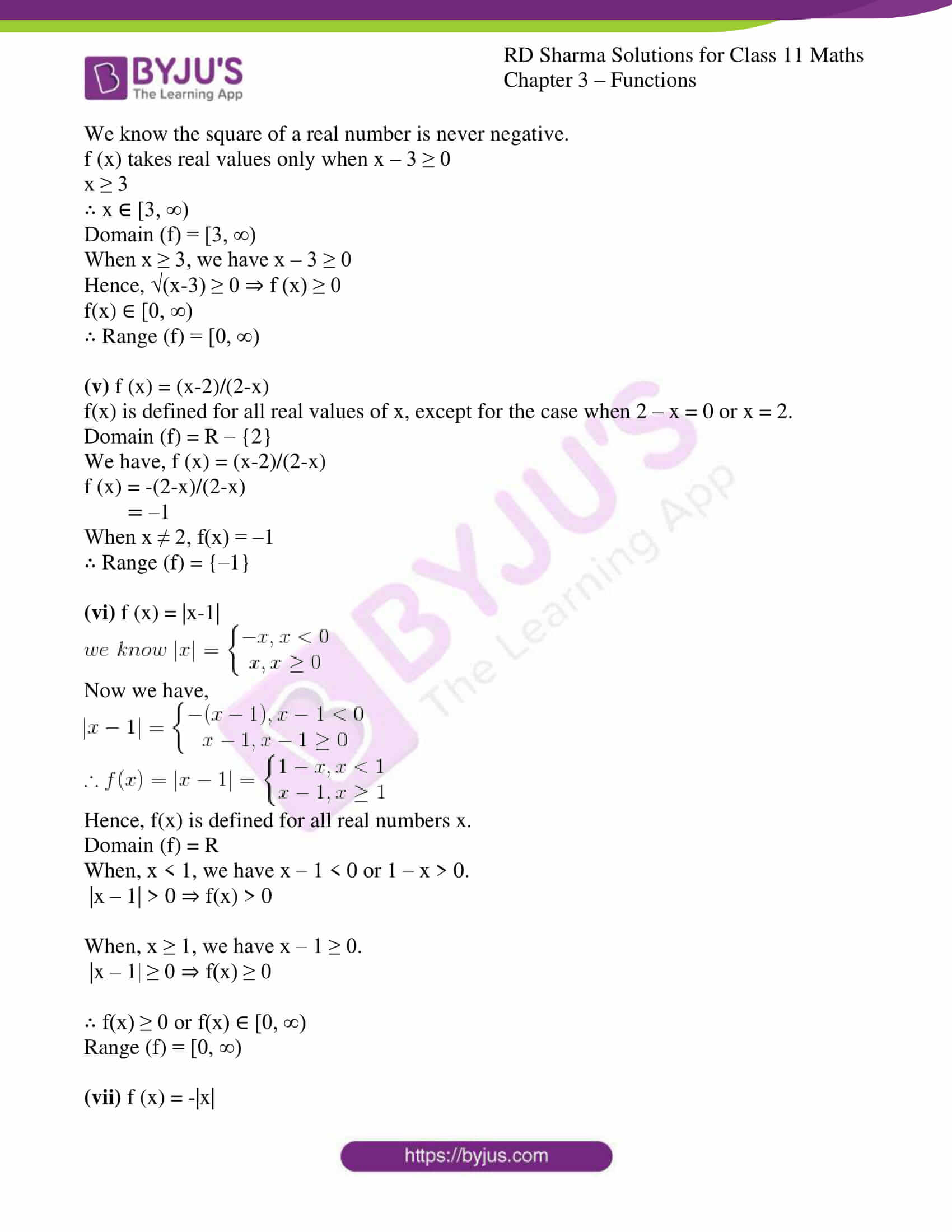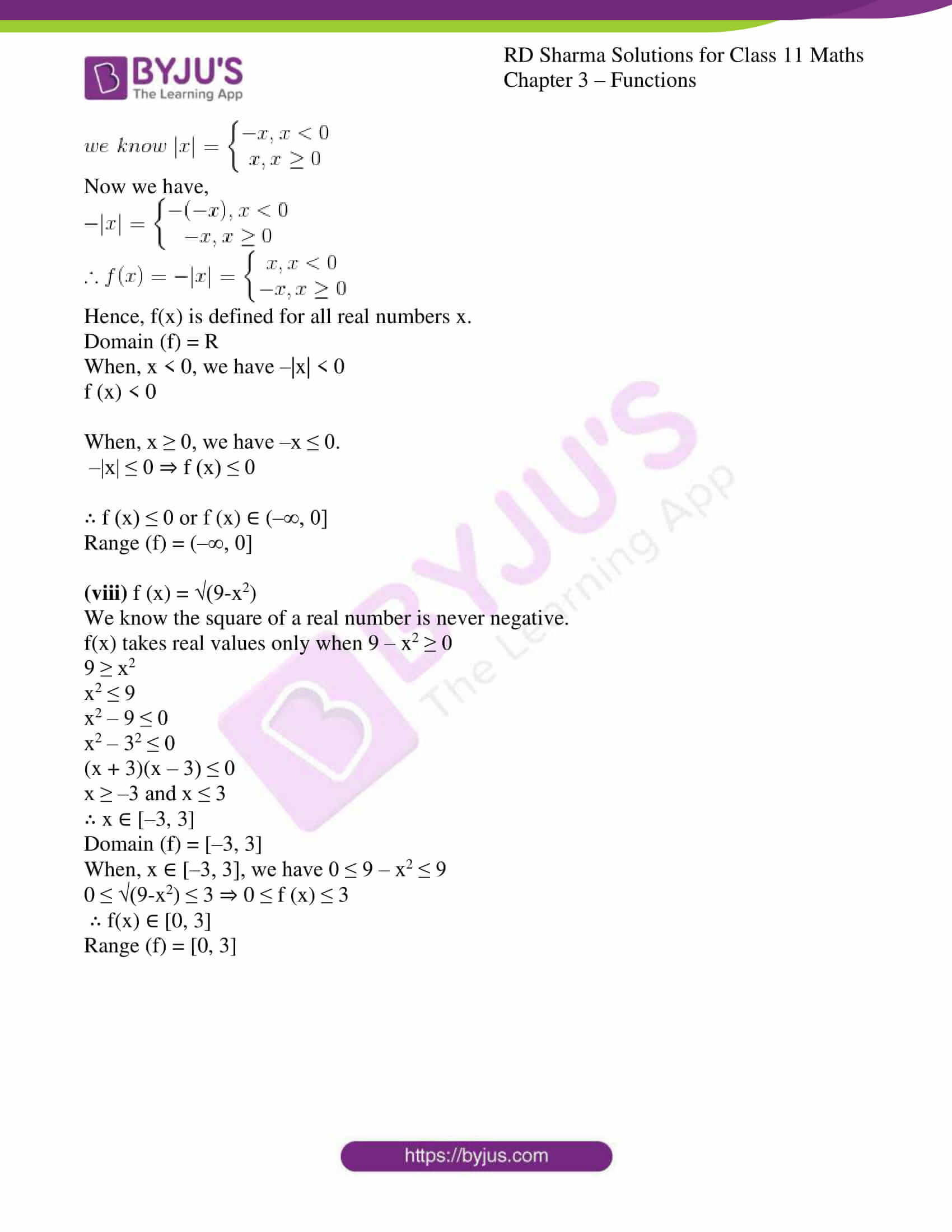### Access answers to RD Sharma Solutions for Class 11 Maths Exercise 3.3 Chapter 3 – Functions

1. Find the domain of each of the following real valued functions of real variable:

(i) f (x) = 1/x

(ii) f (x) = 1/(x-7)

(iii) f (x) = (3x-2)/(x+1)

(iv) f (x) = (2x+1)/(x2-9)

(v) f (x) = (x2+2x+1)/(x2-8x+12)

Solution:

(i) f (x) = 1/x

We know, f (x) is defined for all real values of x, except for the case when x = 0.

∴ Domain of f = R – {0}

(ii) f (x) = 1/(x-7)

We know, f (x) is defined for all real values of x, except for the case when x – 7 = 0 or x = 7.

∴ Domain of f = R – {7}

(iii) f (x) = (3x-2)/(x+1)

We know, f(x) is defined for all real values of x, except for the case when x + 1 = 0 or x = –1.

∴ Domain of f = R – {–1}

(iv) f (x) = (2x+1)/(x2-9)

We know, f (x) is defined for all real values of x, except for the case when x2 – 9 = 0.

x2 – 9 = 0

x2 – 32 = 0

(x + 3)(x – 3) = 0

x + 3 = 0 or x – 3 = 0

x = ± 3

∴ Domain of f = R – {–3, 3}

(v) f (x) = (x2+2x+1)/(x2-8x+12)

We know, f(x) is defined for all real values of x, except for the case when x2 – 8x + 12 = 0.

x2 – 8x + 12 = 0

x2 – 2x – 6x + 12 = 0

x(x – 2) – 6(x – 2) = 0

(x – 2)(x – 6) = 0

x – 2 = 0 or x – 6 = 0

x = 2 or 6

∴ Domain of f = R – {2, 6}

2. Find the domain of each of the following real valued functions of real variable:

(i) f (x) = √(x-2)

(ii) f (x) = 1/(√(x2-1))

(iii) f (x) = √(9-x2)

(iv) f (x) = √(x-2)/(3-x)

Solution:

(i) f (x) = √(x-2)

We know the square of a real number is never negative.

f (x) takes real values only when x – 2 ≥ 0

x ≥ 2

∴ x ∈ [2, ∞)

∴ Domain (f) = [2, ∞)

(ii) f (x) = 1/(√(x2-1))

We know the square of a real number is never negative.

f (x) takes real values only when x2 – 1 ≥ 0

x2 – 12 ≥ 0

(x + 1) (x – 1) ≥ 0

x ≤ –1 or x ≥ 1

∴ x ∈ (–∞, –1] ∪ [1, ∞)

In addition, f (x) is also undefined when x2 – 1 = 0 because denominator will be zero and the result will be indeterminate.

x2 – 1 = 0 ⇒ x = ± 1

So, x ∈ (–∞, –1] ∪ [1, ∞) – {–1, 1}

x ∈ (–∞, –1) ∪ (1, ∞)

∴ Domain (f) = (–∞, –1) ∪ (1, ∞)

(iii) f (x) = √(9-x2)

We know the square of a real number is never negative.

f (x) takes real values only when 9 – x2 ≥ 0

9 ≥ x2

x2 ≤ 9

x2 – 9 ≤ 0

x2 – 32 ≤ 0

(x + 3)(x – 3) ≤ 0

x ≥ –3 and x ≤ 3

x ∈ [–3, 3]

∴ Domain (f) = [–3, 3]

(iv) f (x) = √(x-2)/(3-x)

We know the square root of a real number is never negative.

f (x) takes real values only when x – 2 and 3 – x are both positive and negative.

(a) Both x – 2 and 3 – x are positive

x – 2 ≥ 0

x ≥ 2

3 – x ≥ 0

x ≤ 3

Hence, x ≥ 2 and x ≤ 3

∴ x ∈ [2, 3]

(b) Both x – 2 and 3 – x are negative

x – 2 ≤ 0

x ≤ 2

3 – x ≤ 0

x ≥ 3

Hence, x ≤ 2 and x ≥ 3

However, the intersection of these sets is null set. Thus, this case is not possible.

Hence, x ∈ [2, 3] – {3}

x ∈ [2, 3]

∴ Domain (f) = [2, 3]

3. Find the domain and range of each of the following real valued functions:

(i) f (x) = (ax+b)/(bx-a)

(ii) f (x) = (ax-b)/(cx-d)

(iii) f (x) = √(x-1)

(iv) f (x) = √(x-3)

(v) f (x) = (x-2)/(2-x)

(vi) f (x) = |x-1|

(vii) f (x) = -|x|

(viii) f (x) = √(9-x2)

Solution:

(i) f (x) = (ax+b)/(bx-a)

f(x) is defined for all real values of x, except for the case when bx – a = 0 or x = a/b.

Domain (f) = R – (a/b)

Let f (x) = y

(ax+b)/(bx-a) = y

ax + b = y(bx – a)

ax + b = bxy – ay

ax – bxy = –ay – b

x(a – by) = –(ay + b)

∴ x = – (ay+b)/(a-by)

When a – by = 0 or y = a/b

Hence, f(x) cannot take the value a/b.

∴ Range (f) = R – (a/b)

(ii) f (x) = (ax-b)/(cx-d)

f(x) is defined for all real values of x, except for the case when cx – d = 0 or x = d/c. Domain (f) = R – (d/c)

Let f (x) = y

(ax-b)/(cx-d) = y

ax – b = y(cx – d)

ax – b = cxy – dy

ax – cxy = b – dy

x(a – cy) = b – dy

∴ x = (b-dy)/(a-cy)

When a – cy = 0 or y = a/c,

Hence, f(x) cannot take the value a/c.

∴ Range (f) = R – (a/c)

(iii) f (x) = √(x-1)

We know the square of a real number is never negative.

f(x) takes real values only when x – 1 ≥ 0

x ≥ 1

∴ x ∈ [1, ∞)

Thus, domain (f) = [1, ∞)

When x ≥ 1, we have x – 1 ≥ 0

Hence, √(x-1) ≥ 0 ⇒ f (x) ≥ 0

f(x) ∈ [0, ∞)

∴ Range (f) = [0, ∞)

(iv) f (x) = √(x-3)

We know the square of a real number is never negative.

f (x) takes real values only when x – 3 ≥ 0

x ≥ 3

∴ x ∈ [3, ∞)

Domain (f) = [3, ∞)

When x ≥ 3, we have x – 3 ≥ 0

Hence, √(x-3) ≥ 0 ⇒ f (x) ≥ 0

f(x) ∈ [0, ∞)

∴ Range (f) = [0, ∞)

(v) f (x) = (x-2)/(2-x)

f(x) is defined for all real values of x, except for the case when 2 – x = 0 or x = 2.

Domain (f) = R – {2}

We have, f (x) = (x-2)/(2-x)

f (x) = -(2-x)/(2-x)

= –1

When x ≠ 2, f(x) = –1

∴ Range (f) = {–1}

(vi) f (x) = |x-1|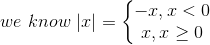Now we have,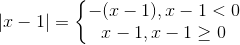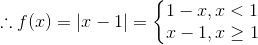Hence, f(x) is defined for all real numbers x.

Domain (f) = R

When, x < 1, we have x – 1 < 0 or 1 – x > 0.

|x – 1| > 0 ⇒ f(x) > 0

When, x ≥ 1, we have x – 1 ≥ 0.

|x – 1| ≥ 0 ⇒ f(x) ≥ 0

∴ f(x) ≥ 0 or f(x) ∈ [0, ∞)

Range (f) = [0, ∞)

(vii) f (x) = -|x|Now we have,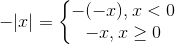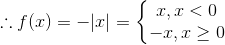Hence, f(x) is defined for all real numbers x.

Domain (f) = R

When, x < 0, we have –|x| < 0

f (x) < 0

When, x ≥ 0, we have –x ≤ 0.

–|x| ≤ 0 ⇒ f (x) ≤ 0

∴ f (x) ≤ 0 or f (x) ∈ (–∞, 0]

Range (f) = (–∞, 0]

(viii) f (x) = √(9-x2)

We know the square of a real number is never negative.

f(x) takes real values only when 9 – x2 ≥ 0

9 ≥ x2

x2 ≤ 9

x2 – 9 ≤ 0

x2 – 32 ≤ 0

(x + 3)(x – 3) ≤ 0

x ≥ –3 and x ≤ 3

∴ x ∈ [–3, 3]

Domain (f) = [–3, 3]

When, x ∈ [–3, 3], we have 0 ≤ 9 – x2 ≤ 9

0 ≤ √(9-x2) ≤ 3 ⇒ 0 ≤ f (x) ≤ 3

∴ f(x) ∈ [0, 3]

Range (f) = [0, 3]